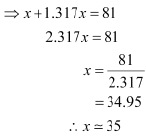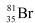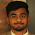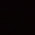## Wednesday, 3 July 2013

### An element with mass number 81 contains 31.7% more neutrons as compared to protons. Assign the atomic symbol.

Q- 2.42 An element with mass number 81 contains 31.7% more neutrons as compared to protons. Assign the atomic symbol.
Let the number of protons in the element be x. ∴ Number of neutrons in the element
= x + 31.7% of x
= x + 0.317 x
= 1.317 x
According to the question,
Mass number of the element = 81
∴ (Number of protons + number of neutrons) = 81Hence, the number of protons in the element i.e., x is 35.
Since the atomic number of an atom is defined as the number of protons present in its nucleus, the atomic number of the given element is 35.
∴ The atomic symbol of the element is1.1.2.Thank you so much

2.This comment has been removed by the author.

3.How do we get 2.317x. ?

1.As we got 1.317x

2.X means1×x.So (1+1.37)x implies or equal to 2.137x

4.How do we get 2.317

1.There is the addition of X and 1.317x, only X means 1x so 1 +1.317 =2.317

5.Thank you sir

6.This comment has been removed by the author.

7.From where we get 0.317

1.31.7% can be converted as that in percentage to (31.7×100=0.317)

8.9.10.thank u thank u thank u so much😍😍😍

11.12.13.Thanks for solution

14.From where we get 31.7 to 0.317

15.How do we get 1.317x